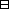## Group Theory and Linear Algebra

Last updated: 21 September 2014

## Lecture 13: Block decomposition

Let $V$ be a vector space. Let $B=\left\{{b}_{1},\dots ,{b}_{k}\right\}$ and $C=\left\{{c}_{1},\dots ,{c}_{\ell }\right\}$ be bases of $V\text{.}$ Then $k=\ell \text{.}$Proof.

Assume $k<\ell \text{.}$
Let $C\prime =\left\{{c}_{2},\dots ,{c}_{\ell }\right\}\text{.}$
Then there exists ${b}_{j}\in B$ such that ${b}_{j}\notin \text{span}\left(C\prime \right)\text{.}$Proof. If all ${b}_{1},\dots ,{b}_{k}\in \text{span}\left(C\prime \right)$ then $span(B)⊆span(C′) andV⊆span(C′).$ Since ${c}_{1}\in V$ then ${c}_{1}\in \text{span}\left(C\prime \right)\text{.}$ So ${c}_{1}={\gamma }_{2}{c}_{2}+\cdots +{\gamma }_{\ell }{c}_{\ell }$ for some ${\gamma }_{2},\dots ,{\gamma }_{\ell }\in 𝔽\text{.}$ So $0=-{c}_{1}+{\gamma }_{2}{c}_{2}+\cdots +{\gamma }_{\ell }{c}_{\ell }\text{.}$ This is a contradiction to the linear independence of $C\text{.}$ So there exists ${b}_{j}$ such that ${b}_{j}\notin \text{span}\left(C\prime \right)\text{.}$ $\square$

Reindex $B$ so that ${b}_{1}\notin \text{span}\left(C\prime \right)$ and let ${C}_{1}=\left\{{b}_{1},{c}_{2},{c}_{3},\dots ,{c}_{\ell }\right\}\text{.}$

Claim ${C}_{1}$ is a basis of $V\text{.}$Proof of claim.

To show:
 (a) ${C}_{1}$ is linearly independent. (b) $\text{span}\left({C}_{1}\right)=V\text{.}$
(a)
 To show: If ${\alpha }_{1}{b}_{1}+{\alpha }_{2}{c}_{2}+\cdots +{\alpha }_{\ell }{c}_{\ell }=0$ then ${\alpha }_{1}=0,$ ${\alpha }_{2}=0,$ $\dots ,$ ${\alpha }_{\ell }=0\text{.}$ Assume ${\alpha }_{1}{b}_{1}+{\alpha }_{2}{c}_{2}+\cdots +{\alpha }_{\ell }{c}_{\ell }=0\text{.}$ Case 1 $\alpha \ne 0\text{.}$ Then ${b}_{1}=-\frac{{\alpha }_{2}}{{\alpha }_{1}}{c}_{2}-\frac{{\alpha }_{3}}{{\alpha }_{1}}{c}_{3}-\cdots -\frac{{\alpha }_{\ell }}{{\alpha }_{1}}{a}_{\ell }\text{.}$ So ${b}_{1}\in \text{span}\left(C\prime \right),$ which is a contradiction to the choice of ${b}_{1}\text{.}$ So ${\alpha }_{1}=0\text{.}$ Case 2 ${\alpha }_{1}=0\text{.}$ Then ${\alpha }_{2}{c}_{2}+\cdots +{\alpha }_{\ell }{c}_{\ell }=0\text{.}$ Since $C$ is linearly independent $α2=0, α3=0,…, αℓ=0.$
(b)
 To show: $V=\text{span}\left\{{b}_{1},{c}_{2},\dots ,{c}_{\ell }\right\}\text{.}$ To show: $V\subseteq \text{span}\left\{{b}_{1},{c}_{2},\dots ,{c}_{\ell }\right\}\text{.}$ To show: $\text{span}\left\{{c}_{1},{c}_{2},\dots ,{c}_{\ell }\right\}\subseteq \text{span}\left\{{b}_{1},{c}_{2},\dots ,{c}_{\ell }\right\}\text{.}$ To show: ${c}_{1}\in \text{span}\left\{{b}_{1},{c}_{2},\dots ,{c}_{\ell }\right\}\text{.}$ Since $C$ is a basis there exist ${\alpha }_{1},\dots ,{\alpha }_{\ell }\in 𝔽$ with ${b}_{1}={\alpha }_{1}{c}_{1}+{\alpha }_{2}{c}_{2}+\cdots +{\alpha }_{\ell }{c}_{\ell }\text{.}$ Case 1 ${\alpha }_{1}=0\text{.}$ Then ${b}_{1}={\alpha }_{2}{c}_{2}+\cdots +{\alpha }_{\ell }{c}_{\ell }$ and ${b}_{1}\in \text{span}\left(C\prime \right)\text{.}$ This is a contradiction to the choice of ${b}_{1}\text{.}$ So ${\alpha }_{1}\ne 0\text{.}$ Case 2 ${\alpha }_{2}\ne 0\text{.}$ Then ${c}_{1}={\alpha }_{1}^{-1}\left({b}_{1}-{\alpha }_{2}{c}_{2}-\cdots -{\alpha }_{\ell }{c}_{\ell }\right)\text{.}$ So ${c}_{1}\in \text{span}\left\{{b}_{1},{c}_{2},\dots ,{c}_{\ell }\right\}\text{.}$
So ${C}_{1}=\left\{{b}_{1},{c}_{2},\dots ,{c}_{\ell }\right\}$ is a basis of $V\text{.}$

$\square$

Let ${C}_{1}^{\prime }=\left\{{b}_{1},{c}_{3},\dots ,{c}_{\ell }\right\}$ and let ${b}_{j}\in B$ such that ${b}_{j}\notin \text{span}\left({C}_{1}^{\prime }\right)\text{.}$
Reindex $B$ so that ${b}_{2}\notin \text{span}\left({C}_{1}^{\prime }\right)\text{.}$
Let ${C}_{2}=\left\{{b}_{1},{b}_{2},{c}_{3},\dots ,{c}_{\ell }\right\}\text{.}$
Then, by a proof as for ${C}_{1}$ above, ${C}_{2}$ is a basis of $V\text{.}$
Continue this process to obtain $Ck= {b1,b2,…,bk,ck+1,ck+2,…,cℓ}$ which is a basis of $V\text{.}$ Since $B$ is a basis of $V,$ then $ck+1=α1b1 +α2b2+⋯+αk bk,$ for some ${\alpha }_{1},\dots ,{\alpha }_{k}\in 𝔽\text{.}$
So $0={\alpha }_{1}{b}_{1}+\cdots +{\alpha }_{k}{b}_{k}-{c}_{k+1}\text{.}$
This contradicts the linear independence of ${C}_{k}\text{.}$
So $k\nless \ell \text{.}$
A similar argument shows $\ell \nless k\text{.}$
So $k=\ell \text{.}$

$\square$

Let $V$ be a vector space and let $f:V\to V$ be a linear transformation. Let $m\left(t\right)$ be the minimal polynomial of $f\text{.}$ Assume that $m(t)=p(t)q(t)$ with $\text{gcd}\left(p,q\right)=1\text{.}$ Let $k\left(t\right)$ and $\ell \left(t\right)$ be such that $1=p(t)k(t) +q(t)ℓ(t).$ Let $U=p(f)k(f)V andW=q(f)ℓ(f) V.$ Then $V=U⊕W.$

Let $A= ( 20 12 30 13 ) .$ The minimal polynomial of $A$ is $m(t)= (t-2)2 (t-3)2$ and $p\left(t\right)={\left(t-2\right)}^{2},$ $q\left(t\right)={\left(t-3\right)}^{2}\text{.}$ $p(t)=t2-4t+4and q(t)=t2-6t+9.$ $t2-4t+4 = (t2-6t+9)+ (2t-5) t2-6t+9 = (2t-5) (12t-74)+14$ since $t2-4t+4= (t2-6t+9)+ (2t-5).$ $12t-74 2t-5 | t2-6t+9 ‾ | t2-52t | t2 -72t+9 ‾ | t2 -72t+354 0.5 | t2 -72t+ 14 ‾$ So $t2-6t+9= (12t-74) (2t-5)+14.$ So $1 = 4(t2-6t+9)- (2t-7)(2t-5) = 4(t2-6t+9)- (2t-7) ((t2-4t+4)-(t2-6t+9)) = (2t-3) (t2-6t+9)- (2t-7) (t2-4t+4) = (2t-3)(t-3)2 -(2t-7)(t-2)2.$ Then $(2A-3) (A-3)2 = ( 10 21 30 23 ) ( -1 1-1 0 10 ) 2 = ( 1 21 3 23 ) ( 10 -21 00 00 ) = ( 10 01 00 00 )$ and $-(2A-7) (A-2)2 = ( 30 -23 10 -21 ) ( 00 10 10 11 ) 2 = ( 30 -23 10 -21 ) ( 00 00 10 21 ) = ( 00 00 10 01 ) .$

## Notes and References

These are a typed copy of Lecture 13 from a series of handwritten lecture notes for the class Group Theory and Linear Algebra given on August 23, 2011.